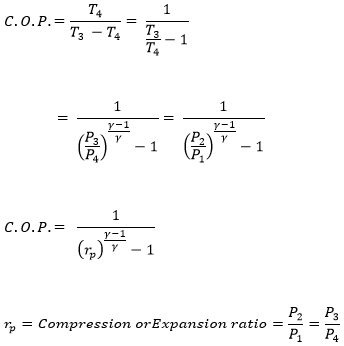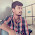# Air Refrigerator Working On Bell-Coleman Cycle with PV and TS Diagram (Reversed Brayton or Joule Cycle)

Fig shows a schematic diagram of Bell-Coleman refrigerator (reverse Brayton or joule cycle). This refrigeration system components consists of a compressor, cooler, Expander, and refrigerator. In this process, heat absorption and rejection follows at the constant pressure; the compression and expansion of process are isentropic.

### Different process in Bell-Coleman refrigeration

Fig show P-V and T-S diagram of bell coleman refrigerator. Here P1, V1, T1, S1 represents the pressure, volume, temperature, entropy of air respectively at point 1. And so on. It represents the corresponding condition of air when it passed through the component.

1-2: Isentropic Compression
The Air drawn from refrigerator to air compressor cylinder where it compressed isentropically (constant entropy). No heat transfer by the air. During compression, the volume decreases while the pressure and temperature of air increases.

2-3: Constant pressure cooling process.
The warm compressed air is then passed through cooler, where it cooled down at constant pressure.
The heat rejected per kg of air during this process is equal to
q2-3 = Cp(T2-T3)

3-4: isentropic expansion
No heat transfer takesplcae. The air expands isentropically in expander cylinder. During expansion, the volume increases, Pressure P3 reduces to P4. (P4 = atmospheric pressure). Temperature also falls during expansion from T3-T4.

4-1: Constant pressure expansion
Heat transfer from the refrigerator to air. The temperature increases from T4 to T1. Volume increases to V4 due to heat transfer. Heat absorbed by air per kg during this process is equal to
q4-1 = Cp(T1-T4)

- COP of Air Refrigerator working on reversed Carnot cycle with PV and Ts diagram
- Mechanism and working of  vapour compression refrigeration system - with PV and TS diagram

### Equation of Coefficient of performance (COP) of Bell Coleman cycle

Heat absorbed during cycle per kg of air q4-1 = Cp(T1-T4)
Heat rejected during cycle per kg of air q2-3 = Cp(T2-T3)
Then the work done per kg of air during the cycle is = Heat rejected – Heat absorbed
= Cp(T2-T3) - Cp(T1-T4)
Coefficient of performance;For isentropic compression process 1-2For isentropic expansion process 3-4Since, P2 = P3 and P1 = P4, therefore from equation (ii) and (iii)Substitute equation (iv) in (i)### 4 Responses

1.2.Great job :)

3.Can i have a solved question of the gas refrigerator bell coleman circle

4.Excellent expansion